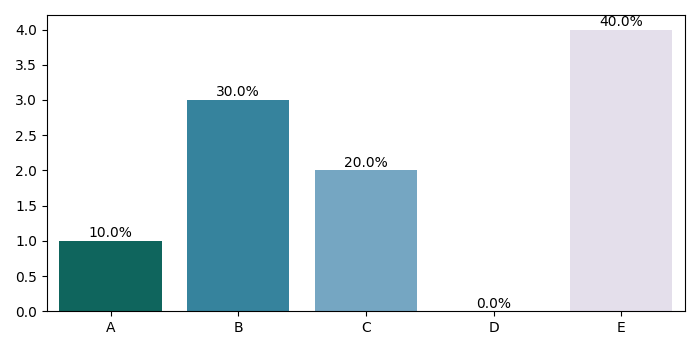# How to add percentages on top of bars in Seaborn using Matplotlib?

To add percentages on top of bars in Seaborn, we can take the following steps −

• Create the lists, x, y and percentages to plot using Seaborn.

• Using barplot, show point estimates and confidence intervals with bars. Store the returned axis.

• Find patches from the returned axis (In step 2).

• Iterate the patches (returned in step 3).

• Find and from the patches to place the percentage value at the top of the bars.

• To display the figure, use show() method.

## Example

import matplotlib.pyplot as plt
import seaborn as sns
plt.rcParams["figure.figsize"] = [7.00, 3.50]
plt.rcParams["figure.autolayout"] = True
x = ['A', 'B', 'C', 'D', 'E']
y = [1, 3, 2, 0, 4]
percentage = [10, 30, 20, 0, 40]
ax = sns.barplot(x=x, y=y, palette='PuBuGn_r')
patches = ax.patches
for i in range(len(patches)):
x = patches[i].get_x() + patches[i].get_width()/2
y = patches[i].get_height()+.05
ax.annotate('{:.1f}%'.format(percentage[i]), (x, y), ha='center')
plt.show()

## Output